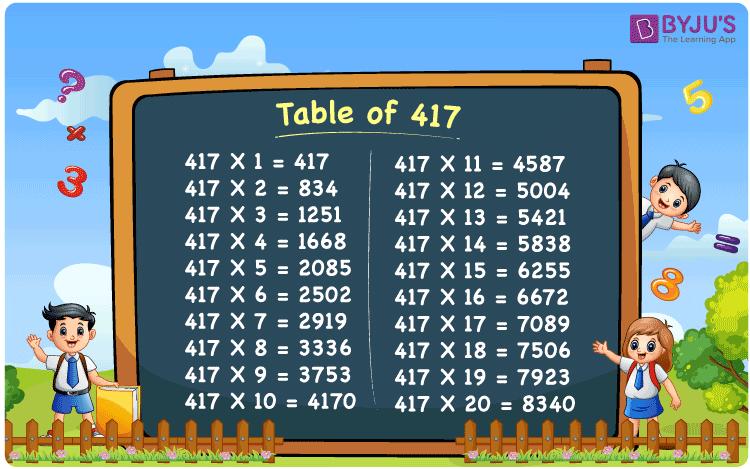Checkout JEE MAINS 2022 Question Paper Analysis : Checkout JEE MAINS 2022 Question Paper Analysis :

# Table of 417

The multiplication table of 417 is available here up to 20 times. The table of 417 can be used to solve mathematical problems in a quick way. 417 times table represents the multiples when 417 is multiplied by a set of natural numbers. It can also be expressed as repeated addition of the original number. For example, 417 x 3 = 417 + 417 + 417 = 1251. Learn more about Multiplication tables at BYJU’S and download the PDF of table 417 here for free.

## Table of 417 Chart## What is 417 Times Table?

The table below shows the repeated addition of the number 417 to get the required multiple.

 417×1 = 417 417 417×2 = 834 417 + 417 = 834 417×3 = 1251 417 + 417 + 417 = 1251 417×4 = 1668 417 + 417 + 417 + 417 = 1668 417×5 = 2085 417 + 417 + 417 + 417 + 417 = 2085 417×6 = 2502 417 + 417 + 417 + 417 + 417 + 417 = 2502 417×7 = 2919 417 + 417 + 417 + 417 + 417 + 417 + 417 = 2919 417×8 = 3336 417 + 417 + 417 + 417 + 417 + 417 + 417 + 417 = 3336 417×9 = 3753 417 + 417 + 417 + 417 + 417 + 417 + 417 + 417 + 417 = 3753 417×10 = 4170 417 + 417 + 417 + 417 + 417 + 417 + 417 + 417 + 417 + 417 = 4170

## Multiplication Table of 417

Here is the table of 417 up to 20 times below.

 417 × 1 = 417 417 × 2 = 834 417 × 3 = 1251 417 × 4 = 1668 417 × 5 = 2085 417 × 6 = 2502 417 × 7 = 2919 417 × 8 = 3336 417 × 9 = 3753 417 × 10 = 4170 417 × 11 = 4587 417 × 12 = 5004 417 × 13 = 5421 417 × 14 = 5838 417 × 15 = 6255 417 × 16 = 6672 417 × 17 = 7089 417 × 18 = 7506 417 × 19 = 7923 417 × 20 = 8340

## Solved Example on Table of 417

Evaluate: 2 x (417 x 3)

Solution: Given,

2 x (417 x 5)

From the table of 417, we have;

417 x 3 = 1251

Therefore,

2 x 1251 = 2502

## Frequently Asked Questions – FAQs

### What is table of 417 in Maths?

In Mathematics, the table of 417 is the multiplication table representing the product of original number with a set of positive integers.

### What is the value of 417 x 9?

The value of 417 times 9 is equal to 3753.

### By what integer should we multiply 417 to get 8340?

By the table of 417, the product of 417 and 20 is equal to 8340.